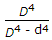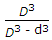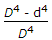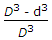# Civil Engineering - Strength of Materials - Discussion

### Discussion :: Strength of Materials - Section 3 (Q.No.1)

1.

The ratio of the moments of resistance of a solid circular shaft of diameter D and a hollow shaft (external diameter D and internal diameter d), is

 [A].[B].[C].[D].[E]. none of these.

Explanation:

No answer description available for this question.

 Taba Amith said: (Jan 27, 2017) The correct answer should be C.

 Ranjitha said: (Mar 29, 2017) I(solid shaft)= π D^4/64. I(hollow shaft)= π (D^4-d^4)/64. So, D^4/(D^4-d^4) is the answer. Option A is correct.

 Priya said: (Nov 18, 2017) Yes, A right. I agree.

 Naveen Kashyap said: (Jan 15, 2018) The answer should be C.

 Deepak said: (Jun 7, 2018) For solid shaft: Tmax = (π/ 16) τmax D3. For a hollow shaft: Tmax = (π/ 16) τmax (D4 - d4)/D.

 Utsav said: (Nov 5, 2018) It's solid to hollow. A is correct.

 Amu Bokato said: (Mar 8, 2019) Thanks all for explaining the answer.

 Nabajit Sarkar said: (Mar 29, 2020) Deflection for UDL = (w/L)*(L^4/8EI). =wL^3/8EI. Deflection of Pt. Load= wL^3/3EI. Max. Deflection = -(5/24)*(wL^3/EI).

 R.K said: (Dec 4, 2020) M= σ *Z = π *d^3* σ /32 (for circular).